﻿ 用射线法定量分析三元相图 The Ray Method Applied to Quantitative Analysis of Ternary Phase Diagram

Material Sciences
Vol.07 No.04(2017), Article ID:21370,9 pages
10.12677/MS.2017.74065

The Ray Method Applied to Quantitative Analysis of Ternary Phase Diagram

Quncheng Fan1*, Jiachen Kang2

1School of Material Science and Engineering, Xi’an Jiaotong University, Xi’an Shaanxi

2School of Material Science and chemical Engineering, Xi’an Technological University, Xi’an Shaanxi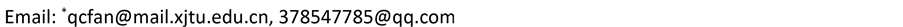Received: Jun. 20th, 2017; accepted: Jul. 11th, 2017; published: Jul. 14th, 2017ABSTRACT

In this paper, a new method-the ray method-was proposed for quantitative analysis of ternary phase diagram, and the brief principle and method of the ray method were introduced. As examples, the crystallization processes of an alloy in two-phase region of isothermal section of W-Cr-Ni ternary phase diagram as well as a high-phosphorus gray cast iron in projection of Fe-C-P ternary phase diagram were quantitatively analyzed by the ray method, and the relative amounts of structural compositions were calculated. The result of the comparison with the microstructure of a high phosphorus gray cast iron showed that the ray method is reliable.

Keywords:Ternary Phase Diagram, Quantitative Analysis, Ray Method

1西安交通大学材料科学与工程学院，陕西 西安

2西安工业大学材料与化工学院，陕西 西安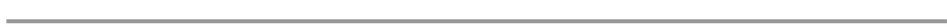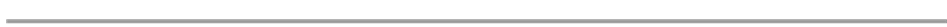1. 引言

2. 射线法的原理简介

2.1. 在二相区的情况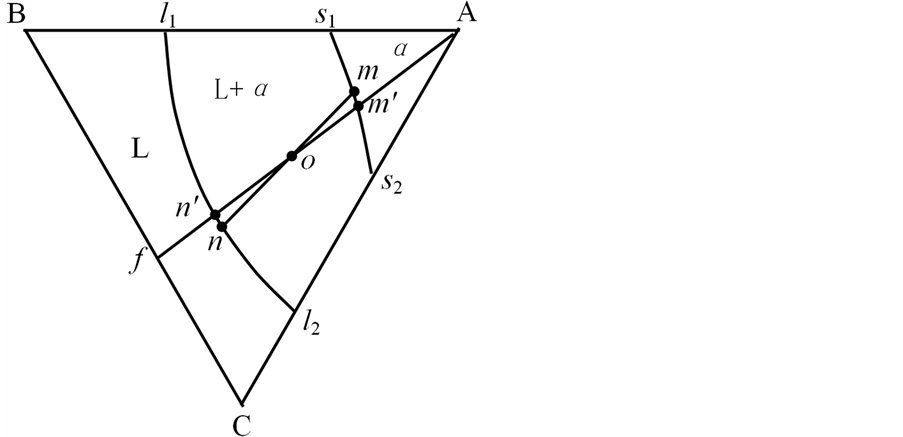Figure 1. Schematic diagram showing an isothermal section of A-B-C ternary phase diagram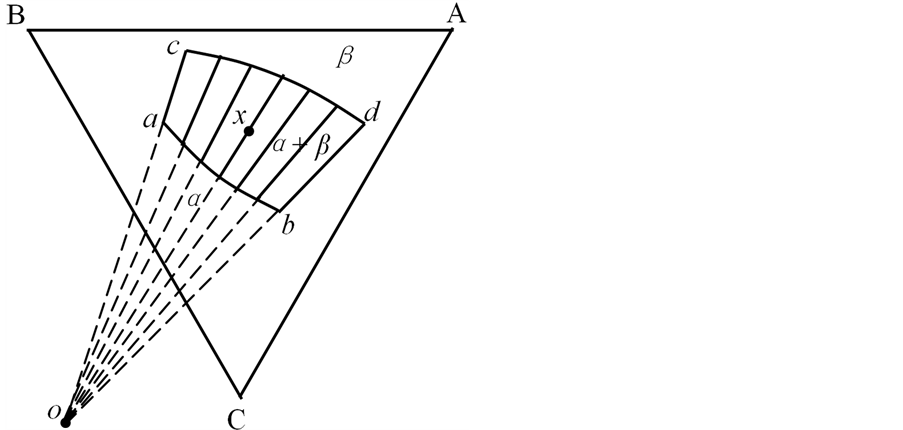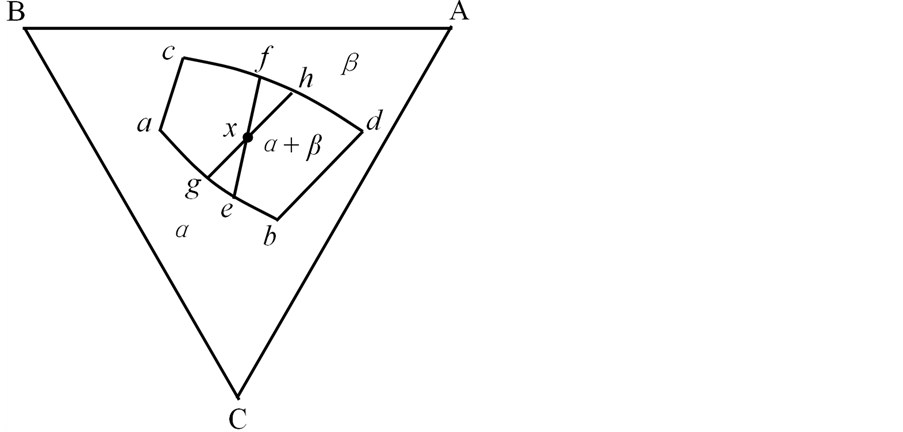(a) (b)

Figure 2. Conjugate connections in two-phase region of isothermal section of ternary phase diagram; (a) Conjugate connections cannot intersect; (b) Conjugate connections can be got by the ray method

2.2. 在三相区的情况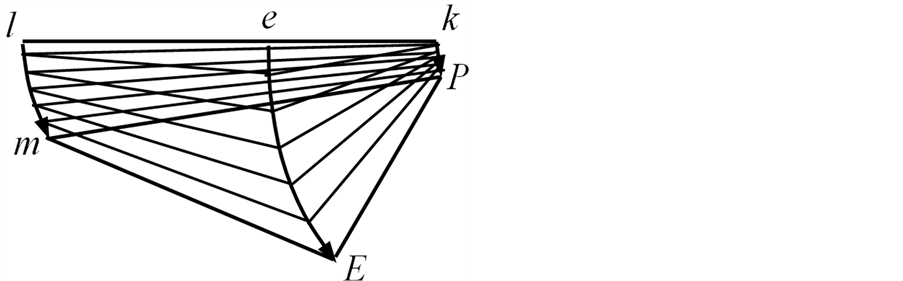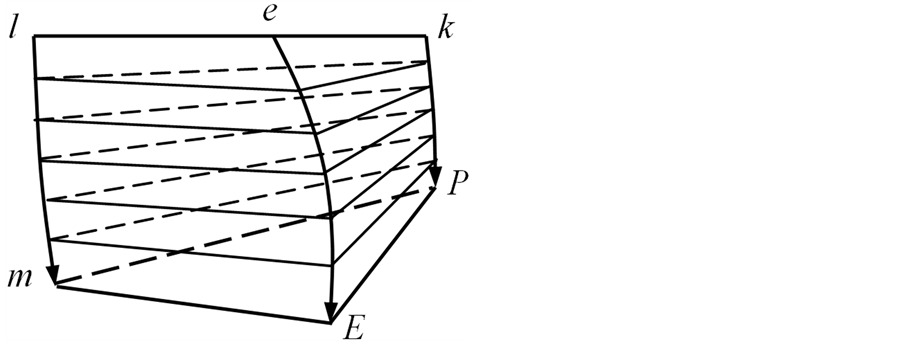(a) (b)

Figure 3. L + α + β three-phase region; (a) Perspective view; (b) Projective viewFigure 4. The L + α + β three-phase region in general projection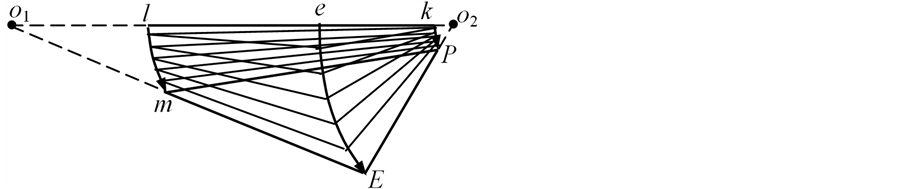Figure 5. Determining the conjugate triangles in projection of L + α + β three-phase area by the ray method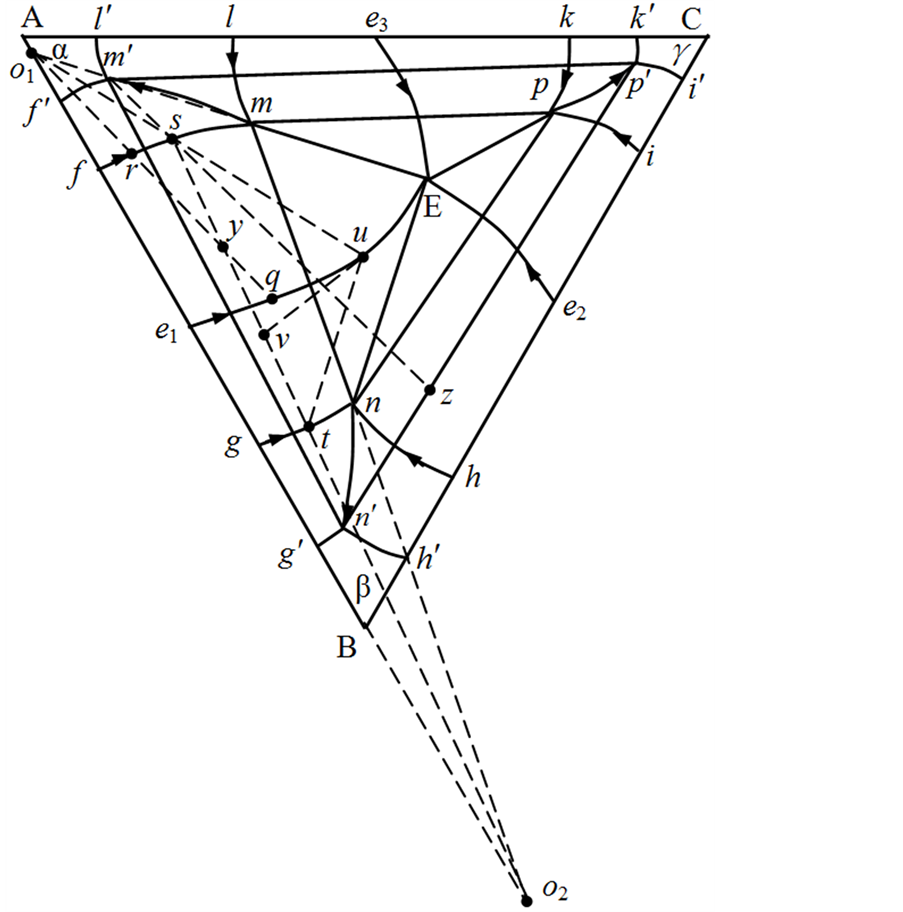Figure 6. Quantitative analysis of solidification process of y alloy in projection of A-B-C ternary phase diagram with four-phase eutectic reaction and limitedly solvated solid phases by the ray method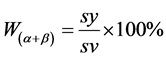(1)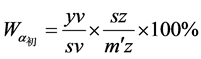(2)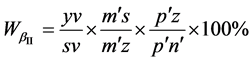(3)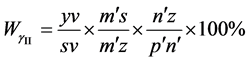(4)

3. 射线法在实际三元相图定量分析中的应用举例

3.1. 在W-Ni-Cr三元相图等温截面中的应用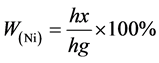(5)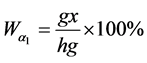(6)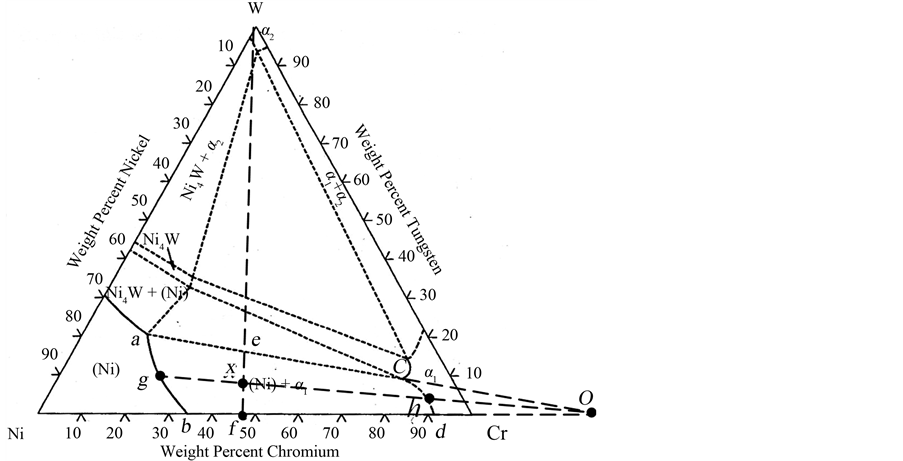Figure 7. Determining the component points g and h of the equilibrium phase of the x alloy in the isothermal section of W-Cr-Ni phase diagram by the ray method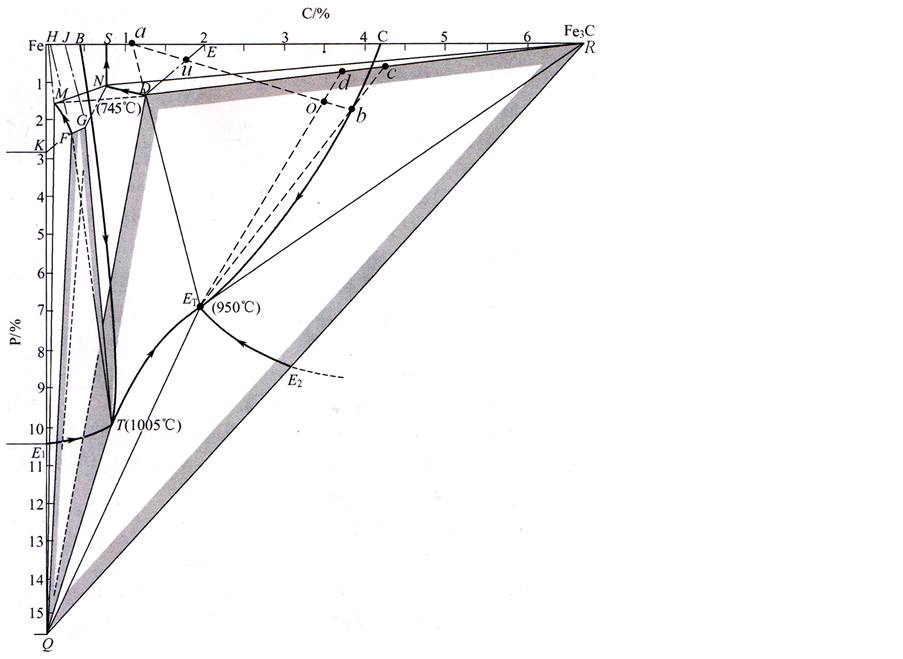Figure 8. Quantitative analysis of solidification process of o alloy in projection of Fe-C-P ternary phase diagram by the ray method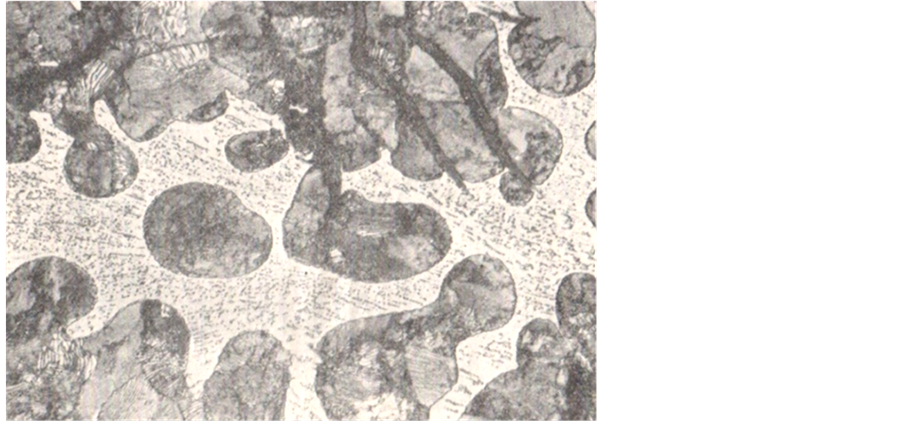Figure 9. The casting microstructure of high-phosphorus gray cast iron with 0.8%P: the network-like ternary phosphorus eutectic is distributed on the fine pearlite matrix, and the black strip is graphite carbon

3.2. 在Fe-C-P三元相图投影图中的应用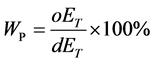(7)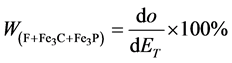(8)

4. 结论

The Ray Method Applied to Quantitative Analysis of Ternary Phase Diagram[J]. 材料科学, 2017, 07(04): 492-500. http://dx.doi.org/10.12677/MS.2017.74065

1. 1. 潘金生, 仝健民, 田民波. 材料科学基础[M]. 北京: 清华大学出版社, 2011: 393.

2. 2. 石德珂, 编. 材料科学基础[M]. 第2版. 北京: 机械工业出版社, 2003: 205.

3. 3. 陶杰, 姚正军, 薛烽,编. 材料科学基础[M]. 北京: 化学工业出版社, 2006: 257.

4. 4. 侯增寿, 陶岚琴, 编. 实用三元合金相图[M]. 上海: 上海科学技术出版社, 1983: 51.

5. 5. 胡赓祥, 蔡珣, 戎咏华, 编. 材料科学基础[M]. 第2版. 上海: 上海交通大学出版社, 2006: 338.

6. 6. 李炯辉, 施友方, 高汉文, 编. 钢铁材料金相图谱[M]. 上海: 上海科学技术出版社, 1981: 84.